## Refrigeration and Air-conditioning Miscellaneous

#### Refrigeration and Air-conditioning

1. A thin layer of water in a field is formed after a farmer has watered it. The ambient air conditions are: temp. 20°C and relative humidity 5%. An extract of steam tables is given below.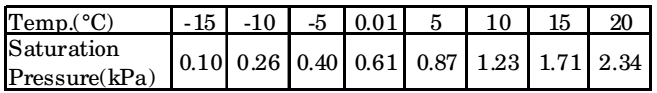Neglecting the heat transfer between the water and the ground, the water temperature in the field after phase equilibrium is reached equals

1. Given data:
Φ = 5% = 0.05
Tdb = 20°C
From table
At 20°C, Ps = 2.34 kPa

 Φ = Pv Ps

 0.05 = Pv 2.34

Pv =.117 k Pa By Interpolation method,
 Tdb = - 15 + (-10 + 15 × (0.117 - .1) 0.26 - 0.10

= – 15 + 0.531 = – 14.5° C

##### Correct Option: C

Given data:
Φ = 5% = 0.05
Tdb = 20°C
From table
At 20°C, Ps = 2.34 kPa

 Φ = Pv Ps

 0.05 = Pv 2.34

Pv =.117 k Pa By Interpolation method,
 Tdb = - 15 + (-10 + 15 × (0.117 - .1) 0.26 - 0.10

= – 15 + 0.531 = – 14.5° C

1. A heat engine having an efficiency of 70% is used to drive a refrigerator having a coefficient of performance of 5. The energy absorbed from low temperature reservoir by the refrigerator for each kJ of energy absorbed from high temperature source by the engine is

1. Given: ηengine = 0.7, (C.O.P)R = 5

 ηengine = W ...(i) Q1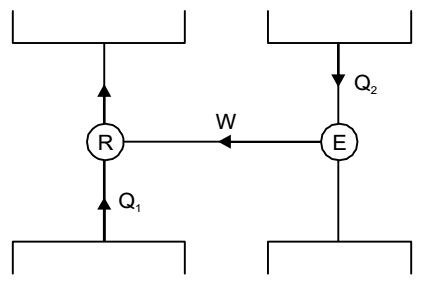Now,
 (C.O.P)R = Q2 W

 ∴ W = Q2 ...(ii) 5

Again,
 0.7 = Q2 × 1 5 Q1

 or Q2 = 3.5 Q1

Energy absorbed from low temperature reservoir by the refrigerator for each kJ of energy absorbed from high temperature source by the engine = 3.5 kJ.

##### Correct Option: C

Given: ηengine = 0.7, (C.O.P)R = 5

 ηengine = W ...(i) Q1Now,
 (C.O.P)R = Q2 W

 ∴ W = Q2 ...(ii) 5

Again,
 0.7 = Q2 × 1 5 Q1

 or Q2 = 3.5 Q1

Energy absorbed from low temperature reservoir by the refrigerator for each kJ of energy absorbed from high temperature source by the engine = 3.5 kJ.

1. The thermodynamic cycle shown in figure (T-s diagram) indicates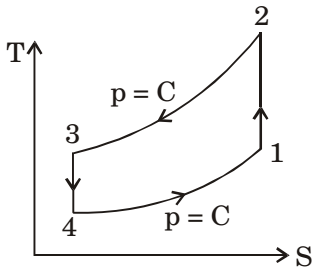1. T-S diagram represent a reversed brayton cycle used in air conditioning of aeroplanes where air is used as a refrigerant.

##### Correct Option: B

T-S diagram represent a reversed brayton cycle used in air conditioning of aeroplanes where air is used as a refrigerant.

1. The COP of a Carnot heat pump operating between 6°C and 37°C is

1.  (COP)c.p. = T1 = 310 = 10 T1 - T2 31

##### Correct Option: A

 (COP)c.p. = T1 = 310 = 10 T1 - T2 31

1. A heat pump with refrigerant R22 is used for space heating between temperature limits of – 20°C and 25°C. The heat required is 200 MJ/ h. Assume specific heat of vapour at the time of discharge as 0.98 kJ/kgK. Other relevant properties are given below. The enthalpy (in kJ/ kg) of the refrigerant at isentropic compressor discharge is_________.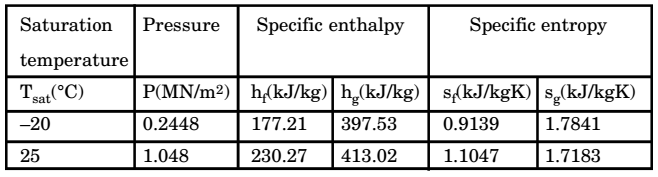1.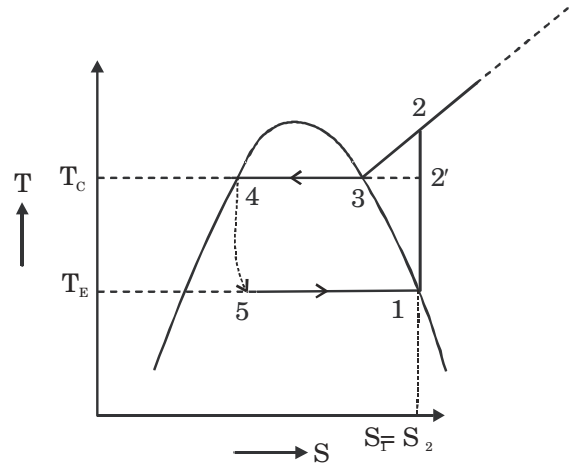Given :T1' = 253 K
T2' = 298 K
S1 = S2 = S2'

 ∴ S1 = S2 = S2' + CP ln T2 T2'

 1.7841= 1.7183 + 0.98 ln T2 298

∴ T2 = 318.69 K
∴ Enthalpy of discharge of compressor
⇒ h2 = h2' + Cp (T2' – T1')
∴ h2 = 413.02 + 0.98 (318.69 – 298)
∴ h2 = 433.3 kJ/kg .

##### Correct Option: AGiven :T1' = 253 K
T2' = 298 K
S1 = S2 = S2'

 ∴ S1 = S2 = S2' + CP ln T2 T2'

 1.7841= 1.7183 + 0.98 ln T2 298

∴ T2 = 318.69 K
∴ Enthalpy of discharge of compressor
⇒ h2 = h2' + Cp (T2' – T1')
∴ h2 = 413.02 + 0.98 (318.69 – 298)
∴ h2 = 433.3 kJ/kg .